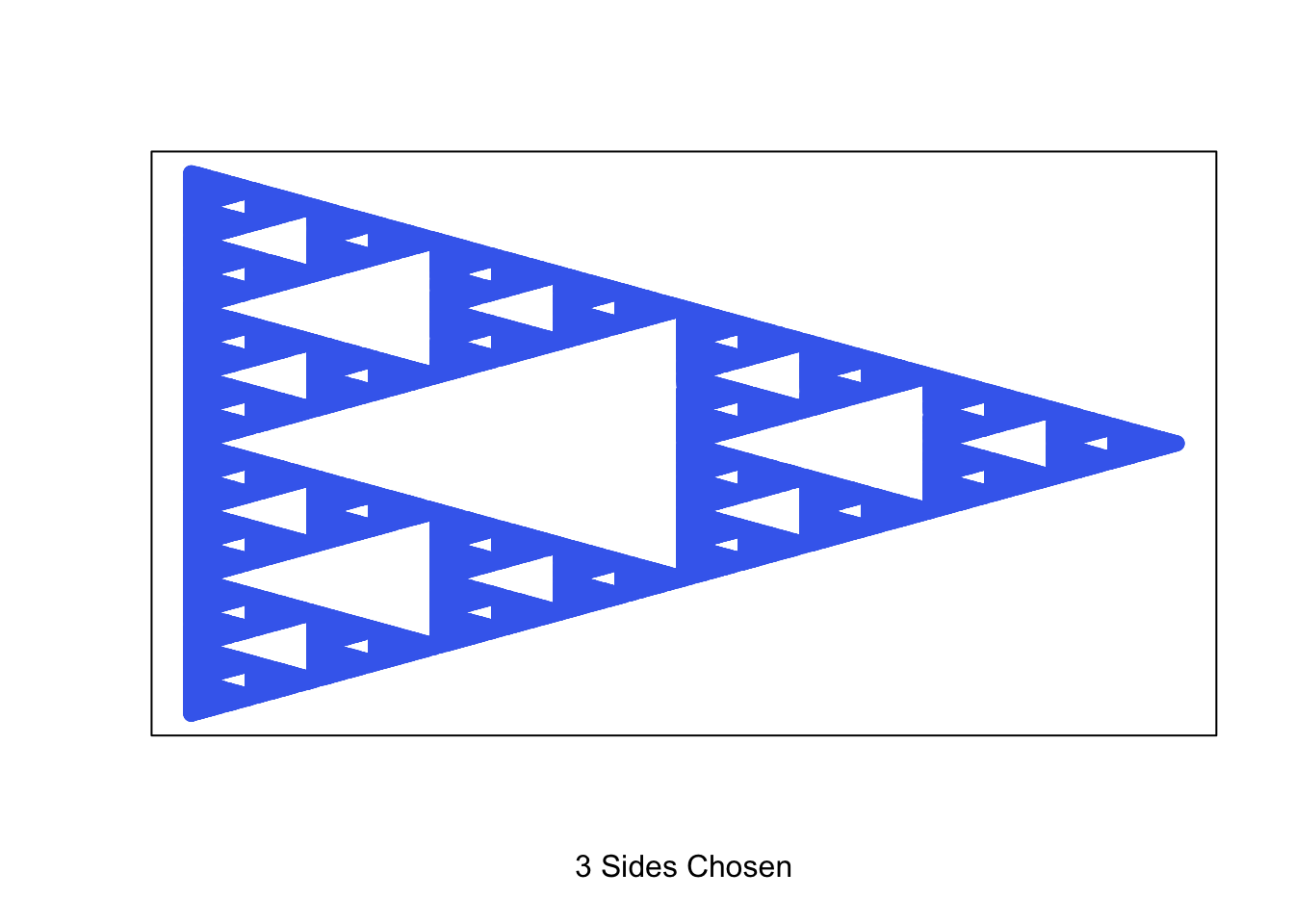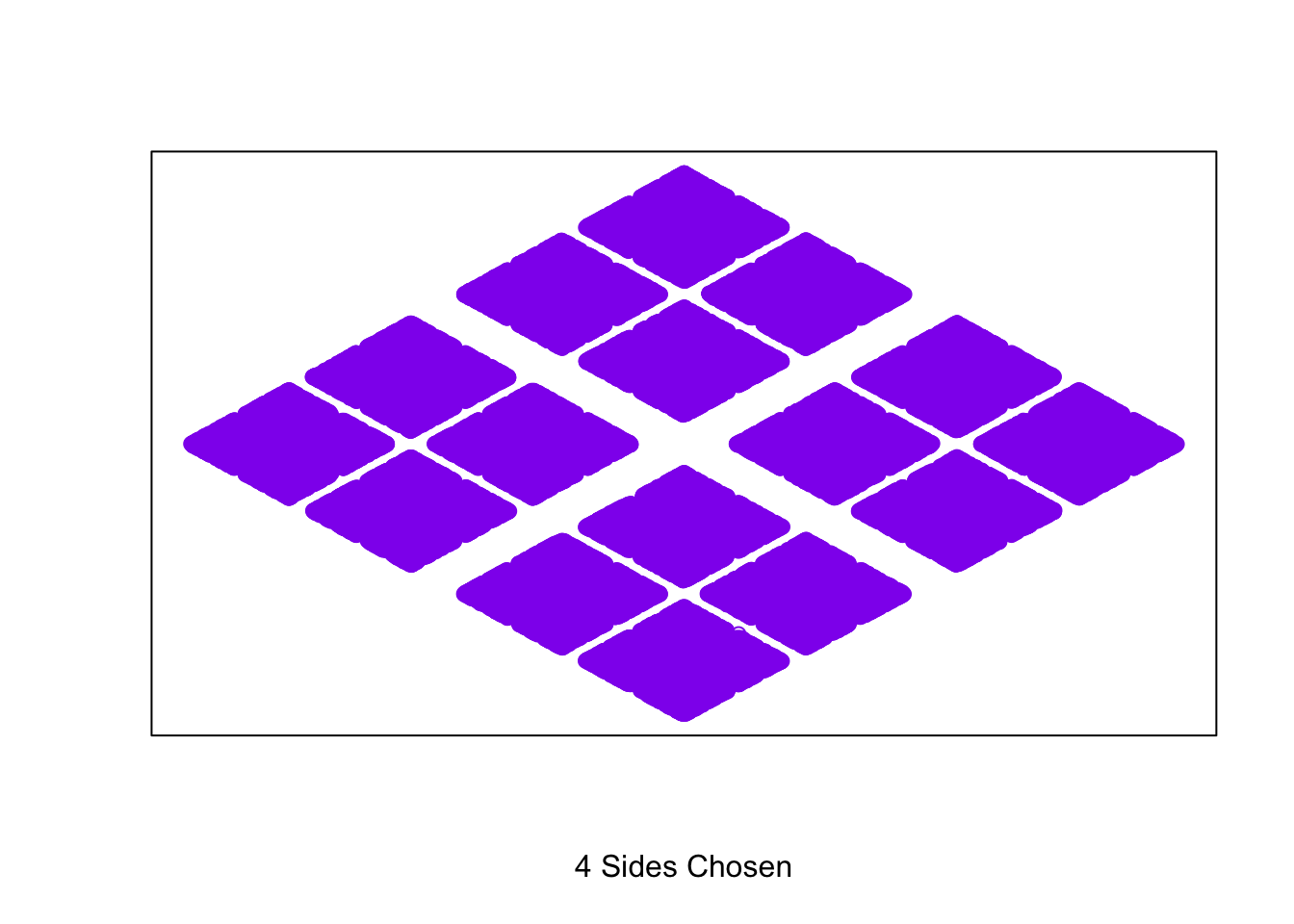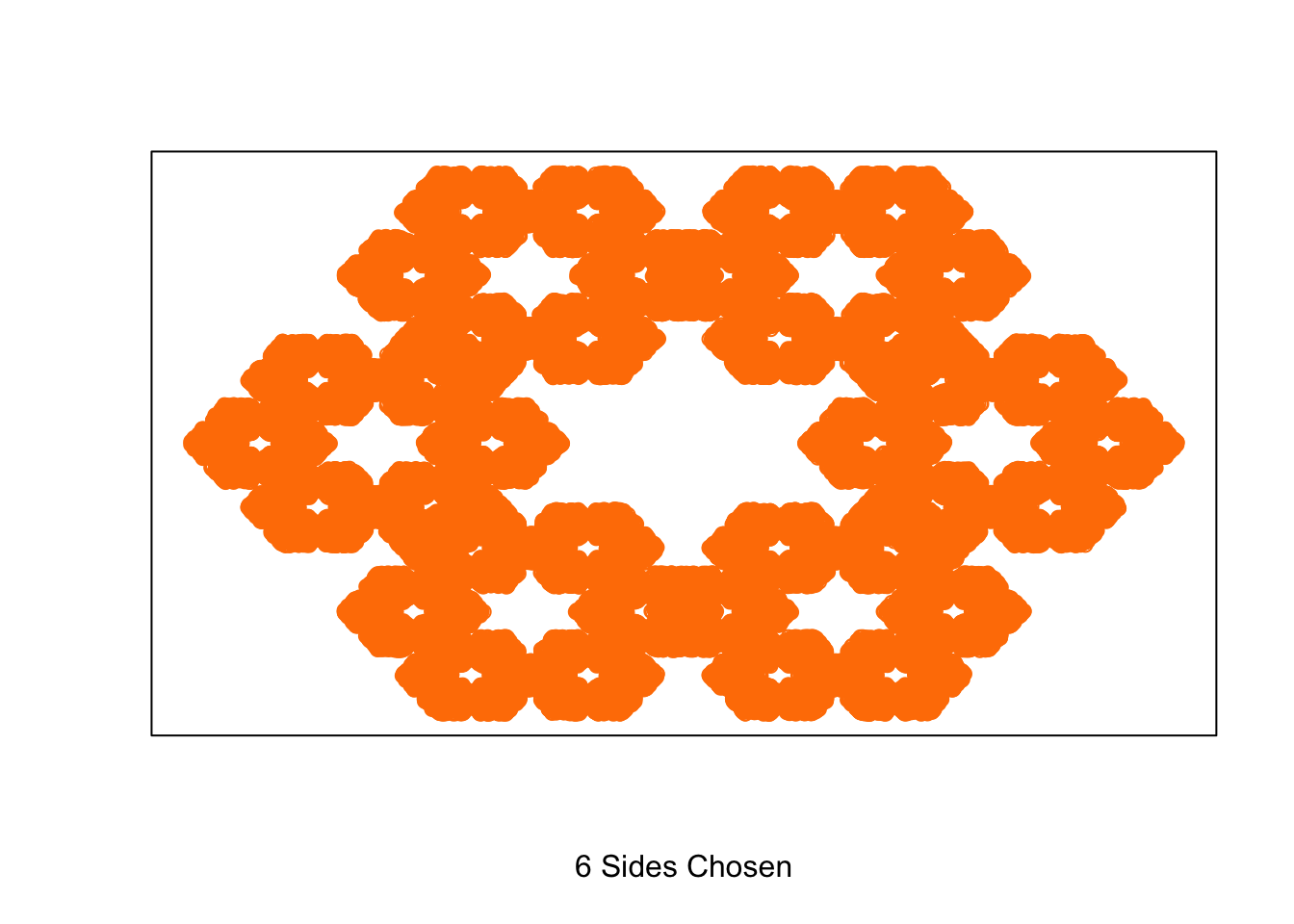# recursive shape fractal generator

Stomperusa · 2019/08/20 · 1 minute read

### Intro

This function allows us to generate the Sierpinski Triangle and explore other recursive shapes with equal length sides following the same algorithm.

shape <- function(corners, trials = 100000){

corners <- as.integer(corners)
points <- list()

if (corners < 3) stop("Value should be 3 or greater")

for (n in 1:(corners)){
points$x[n] <- 0 + cos((2*pi*n)/corners) points$y[n] <- 0 + sin((2*pi*n)/corners)
}

x <- points$x y <- points$y

trials <- trials
sierpinski <- list()

for (t in 1:trials){
r <- sample(1:corners,1)
x <- (x + points$x[r]) / sqrt(corners + 1) y <- (y + points$y[r]) / sqrt(corners + 1)
sierpinski$x[t] <- x sierpinski$y[t] <- y
}

# I use these colors for random color selection. Update for your own desired selection.
color <- sample(c("royalblue2", "firebrick2", "gold2", "springgreen3", "purple2", "darkorange1"),1)

plot(sierpinski$x[corners:trials], sierpinski$y[corners:trials],
xlab = paste0(corners, " Sides Chosen"), ylab = "", xaxt = "n", yaxt = "n", col = color)

}

When you run the function, you indicate the number of sides for the polygon and adjust the number of trials to change the resolution if desired. Here are some examples:

shape(3)shape(4)shape(6)